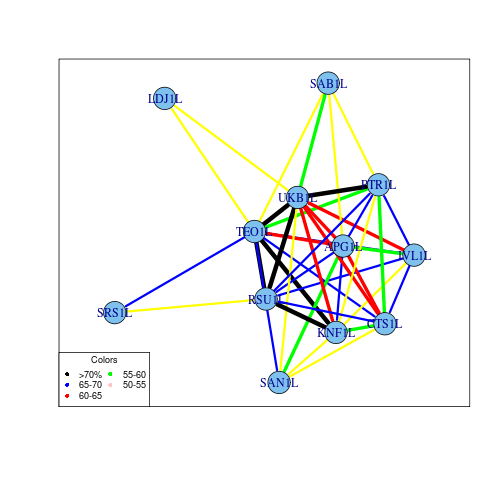Want to share your content on R-bloggers? click here if you have a blog, or here if you don't.

I came up with an idea to draw correlation network to get a grasp about relationship between a list of stocks. An alternative way to show correlation matrix would be head map, which can have limitations with big matrices (>100).
Unfortunately,  ggplot2 package doesn’t have a easy way to draw the networks, so I was left with igraph or network. I tried both, but somehow chose igraph. If you want to master either package I highly recommend to start from theoretical part – it is very well written and it will save your time trying to understand the package’s conception.

Here is correlation matrix of stocks, which correlation coef. is more than 0.5:?View Code RSPLUS
 ```require(xts) require(quantmod) require(igraph) cor_mat<- matrix( runif(100), nr=10 ) cor_mat[ lower.tri(cor_mat, diag=TRUE) ]<- 0 cor_mat[ abs(cor_mat) < 0.5]<- 0   graph <- graph.adjacency(cor_mat>0.5, weighted=TRUE, mode="upper") E(graph)\$weight<-t(cor_mat)[abs(t(cor_mat))>0.5] E(graph)[ weight>0.7 ]\$color <- "black" E(graph)[ weight>=0.65 & weight<0.7 ]\$color <- "red" E(graph)[ weight>=0.6 &weight<0.65 ]\$color <- "green" E(graph)[ weight>=0.55 &weight<0.6 ]\$color <- "blue" E(graph)[ weight<0.55 ]\$color <- "yellow" V(graph)\$label<- seq(1:10)#V(graph)\$name graph\$layout <- layout.fruchterman.reingold factor<-as.factor(cut(E(graph)\$weight*10,c(4,5,6,7,8),labels=c(1,10,20,30))) png('corr_network.png',width=500) plot(decompose.graph(graph)[[which.max(sapply(decompose.graph(graph), vcount))]],edge.width =as.numeric(factor)*1.5,frame=T) legend("bottomleft", title="Colors", cex=0.75, pch=16, col=c("black", "blue","red", "green","pink"), legend=c(">70%", "65-70","60-65","55-60","50-55"), ncol=2) dev.off()```

The code can be found on github.